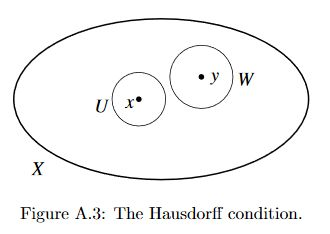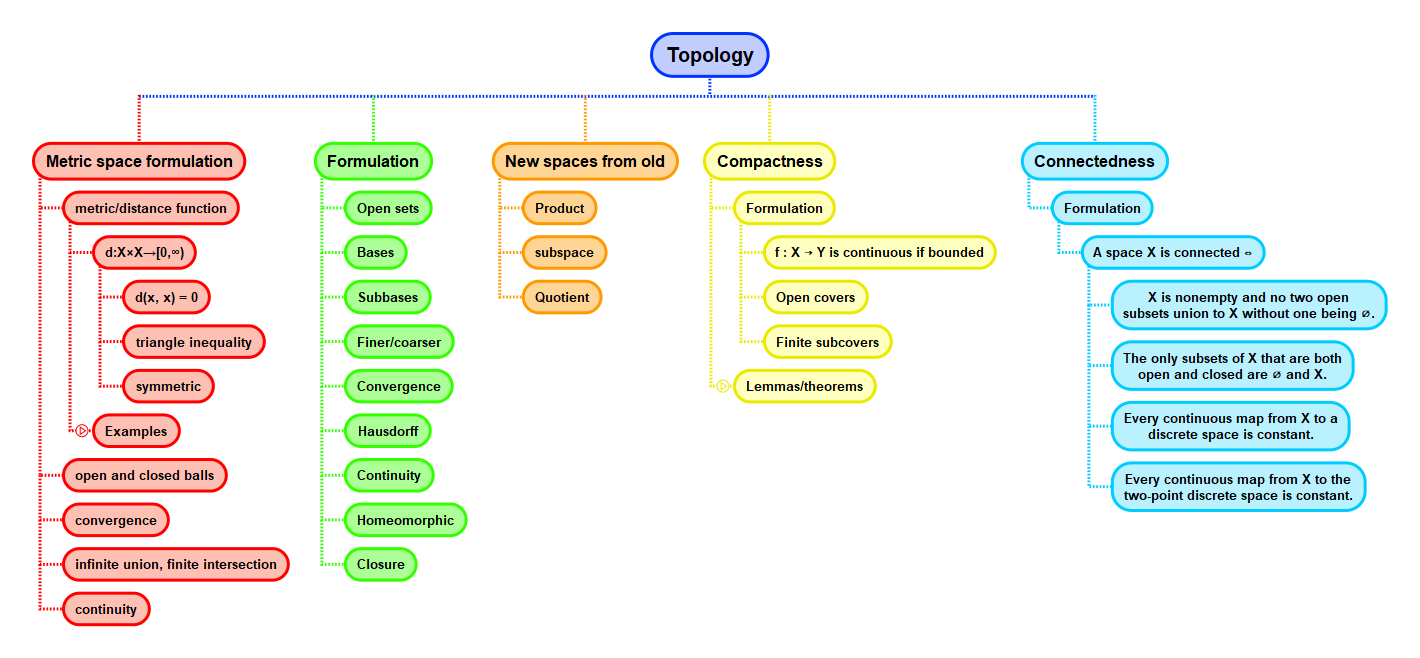Show Question
Math and science::Topology

# Hausdorff spaces

Most interesting topological spaces are Hausdorff. Hausdorffness is identified as being the second separation condition. The first condition, T1 is a sub-requirement of T2 (Housdorff), so it is useful to keep it in mind when thinking about the Hausdorff condition.

### T1

A topological space $$X$$ is said to be $$T_1$$ iff every one-element subset of $$X$$ is closed.

Now for Hausdorff.

### Hausdorff

A topological space $$X$$ is Hausdorff (or $$T_2$$) iff for every distinct $$x, y \in X$$, there exists disjoint neighbourhoods of $$x$$ and $$y$$.

Lemma. Every Hausdorff space is $$T_1$$.

Slightly more precise wording of the Hausdorff condition:

A topological space $$X$$ is Hausdorff (or $$T_2$$) iff for every $$x, y \in X$$ such that $$x \ne y$$, there exists disjoint open sets $$U, W$$ of $$X$$ such that $$x \in U$$ and $$y \in W$$.### Example

Every metrizable space is Hausdorff.

While most interesting spaces are Hausdorff, there are some non-Hausdorff spaces that are important. The Zariski topology is an example.

## Context